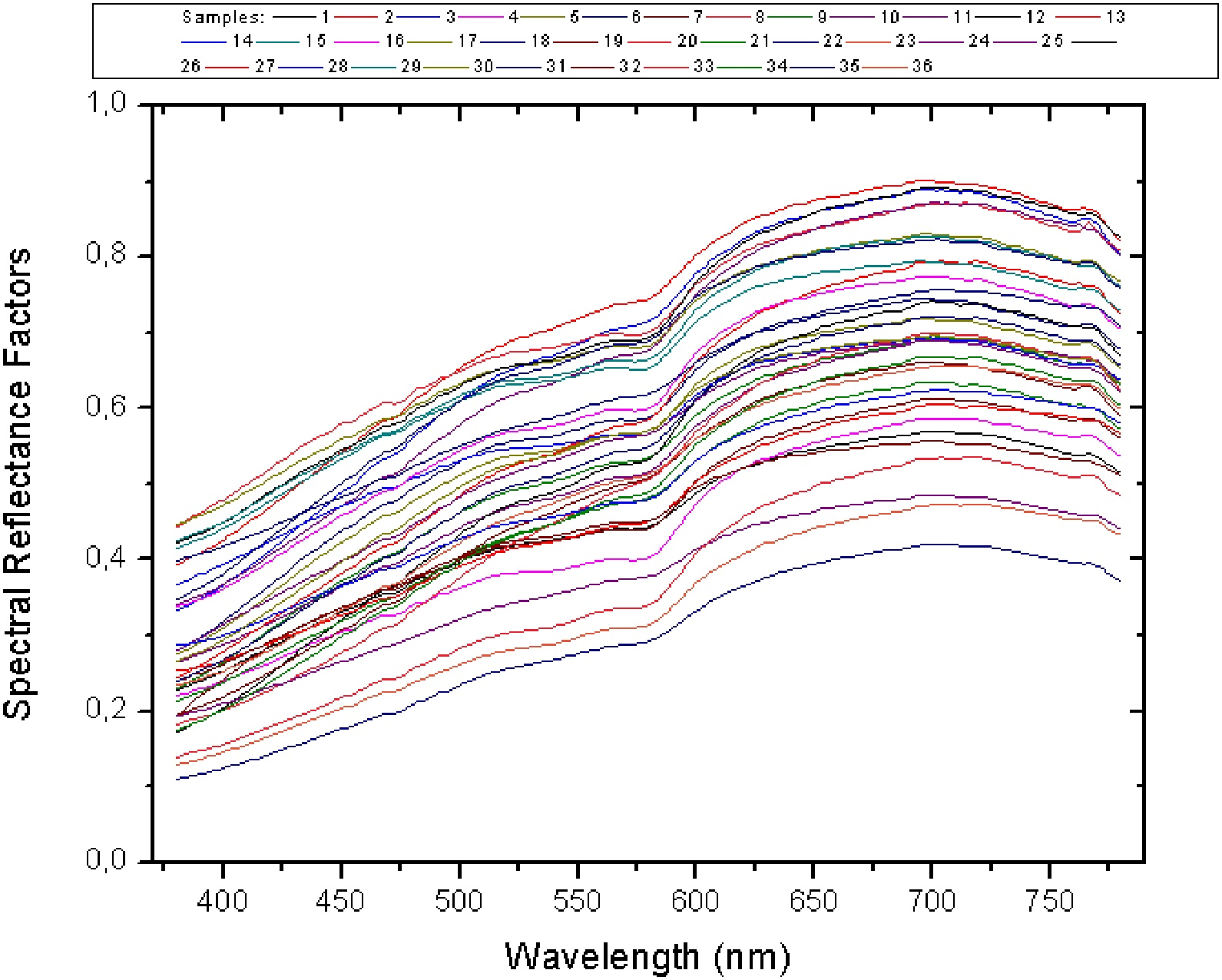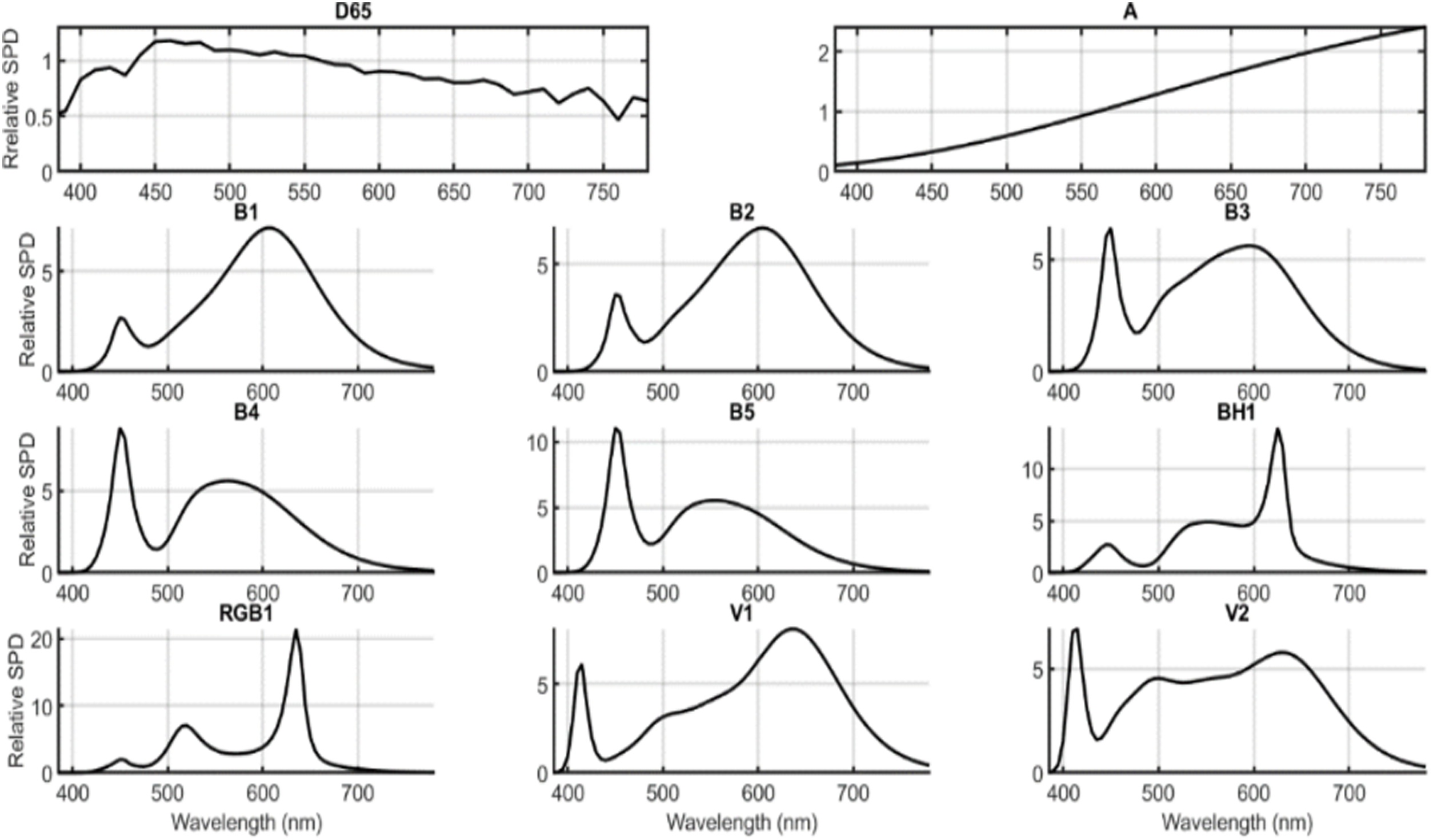# Color inconstancy of natural teeth measured under white light-emitting diode illuminants

## Highlights

• Modern LED sources produce changes in color and whiteness of natural teeth.

• Some LED illuminants recommended by CIE generate perceptible color shifts.

• LED illuminants produce color shifts in very different directions of color space.

• Color differences for pairs of teeth are almost independent of illuminants.

• Whiteness differences for pairs of teeth are almost independent of illuminants.

## Objective

To evaluate color and whiteness changes of natural teeth under nine white LED illuminants recently proposed by CIE.

## Methods

From spectral reflectance factors of 36 caries-free upper central incisors, corresponding colors under illuminant D65 and CIE 1931 standard colorimetric observer were computed for all tested illuminants, using the chromatic adaptation transform CAT16. Color differences (CIELAB and CIEDE2000 units) and whiteness ( <SPAN role=presentation tabIndex=0 id=MathJax-Element-1-Frame class=MathJax style="POSITION: relative" data-mathml='WID’>𝑊𝐼𝐷WID
W I D
and <SPAN role=presentation tabIndex=0 id=MathJax-Element-2-Frame class=MathJax style="POSITION: relative" data-mathml='WIO’>𝑊𝐼𝑂WIO
W I O
indices) from teeth illuminated by CIE standard illuminants (D65 and A) and white LED illuminants were calculated. The appearance of 630 pairs of teeth under all illuminants was also evaluated.

## Results

Color gamuts from 36 teeth under tested illuminants showed similar volume and shape in CIELAB color space, but their centers of gravity changed in many different directions with respect to colors under D65 or A illuminants: Considering colors under D65/A, color shifts produced by white LED illuminants were in the ranges 2.0–8.6/1.1–7.0 CIELAB units (1.4–8.2/0.6–6.8 CIEDE2000 units). Average <SPAN role=presentation tabIndex=0 id=MathJax-Element-3-Frame class=MathJax style="POSITION: relative" data-mathml='WID’>𝑊𝐼𝐷WID
W I D
and <SPAN role=presentation tabIndex=0 id=MathJax-Element-4-Frame class=MathJax style="POSITION: relative" data-mathml='WIO’>𝑊𝐼𝑂WIO
W I O
values for the 36 teeth under different illuminants ranged from −5.8 to +19.3 and from −7.7 to +11.1, respectively. Considering 630 pairs of teeth, average color differences (mainly lightness differences) were below 0.5 CIELAB units (0.3 CIEDE2000 units), and average whiteness differences ranged from 8.1 to 10.7 for <SPAN role=presentation tabIndex=0 id=MathJax-Element-5-Frame class=MathJax style="POSITION: relative" data-mathml='WID’>𝑊𝐼𝐷WID
W I D
(23.4–25.1 for <SPAN role=presentation tabIndex=0 id=MathJax-Element-6-Frame class=MathJax style="POSITION: relative" data-mathml='WIO’>𝑊𝐼𝑂WIO
W I O
).

## Significance

Using CIE LED illuminants, most changes in color and whiteness for individual teeth were above typical threshold values of perceptibility and acceptability in dentistry. However, considering pairs of teeth, the average color and whiteness differences under all tested illuminants were very similar.

## Introduction

There is a current trend to replace traditional light sources for solid-state light sources, mainly light-emitting diodes (LEDs), in most lighting applications [ ]. Despite their popular advantages, like low cost and high energy efficiency, modern LEDs also present a specific caveat, which is their influence on the visual appearance of everyday objects. This is mainly due to the relatively narrow band peaks in their spectral power distribution (SPD), a feature that differs significantly from the SPDs of traditional light sources, like CIE standard illuminants D65 and A, which are considered to be the principal representatives of outdoor and indoor light, respectively. They present relatively smooth SPDs, which are very different from those of modern LED sources. Recently, the Commission Internationale de l’Éclairage (CIE) has proposed a specific set of nine white LED illuminants [ ], to serve as an approximate representation of the range of available white LED sources. This should assist researchers and manufacturers for the analyses of different visual and non-visual effects of white LED sources. The SPDs of these nine LED illuminants were obtained from experimental measurements of 1298 commercial white LED sources [ ]. Specifically, LEDs B1 to B5 (designated as LED-B1 to LED-B5 [ ]) represent typical phosphor-converted blue LEDs with different correlated color temperatures (CCTs), which are nowadays the white LEDs most commonly used. The illuminant BH1 (LED-BH1 [ ]) is a blue-hybrid LED representative for white LEDs that consist of a mixing of phosphor-converted blue and red LEDs. The illuminant RGB1 (LED-RGB1 [ ]) represents typical spectra shapes for mixing of red, green and blue LEDs. Finally, illuminants V1 and V2 (LED-V1 and LED-V2 [ ]) represent two typical phosphor-converted violet LEDs with different CCTs. The main colorimetric characteristics from these nine LED illuminants were previously reported [ ]. For example, these LED illuminants have CCTs in the range 2724 K–6598 K. Currently, simulators of LED illuminants RGB1, V1 and V2 are not often used for white LED lamps. However, multi-channel LED illumination systems, simulating LED illuminants B1 to B5, are currently available in the market [ ].

The influence of these nine CIE white LED illuminants [ ] (hereafter designated as ‘LED illuminants’) on visual appearance has been investigated for a few applications related to cosmetics [ ] and lighting in museums [ ]. The color difference of a single object across two different viewing conditions (e.g. two different illuminants) is defined as ‘color inconstancy’ [ ]. Therefore, the objective of this study was to evaluate the color and whiteness changes [ ] of human teeth as a function of illumination with nine CIE LED illuminants, relative to their color and whiteness under the CIE standard illuminants, D65 and A. Currently, the CIE standard illuminant D65 is recommended for visual color measurements in dentistry [ ], and the CIE standard illuminant A is recommended as representative of indoor lighting.

## Samples and illuminants

A calibrated spectroradiometer (SpectraScan® PR-670, Photo Research Inc., USA) was used to perform in vivo measurements of spectral reflectance factors (380–780 nm in steps of 2 nm) of 36 caries-free teeth (middle third of upper central incisors) in normal occlusion, from 20 Spanish people, 18–30 years old. The spectroradiometric measurements were performed in a dark room where teeth were illuminated by a fiber-coupled Xe-Arc light source (Oriol Research, Newport Corporation, USA), using a calibrated reflectance standard (SRS-3, PhotoResearch, USA) as reference white. The distance between the spectroradiometer and target tooth was 40 cm, approximately. Teeth were irradiated at 45° to the normal and measured at 0° to the normal (i.e. CIE geometry 45°:0°). The measured spectral reflectance factors of 36 teeth are shown in Fig. 1 . The measured spectral reflectance factors of 36 teeth shown in Fig. 1 , suggest a white-yellowish appearance under neutral illumination with relative differences in lightness, as will be discussed latter.Fig. 1

From spectral reflectance factors in Fig. 1 , CIE tristimulus values for illuminants D65, A, and nine LED illuminants ( Fig. 2 ) were computed, assuming the CIE 1931 standard colorimetric observer. It is important to note that direct comparisons among these computed tristimulus values are not adequate because they were obtained under different viewing conditions (i.e. different illuminants). In this case, we must use a ‘chromatic adaptation transform’ [ ] to compute ‘corresponding colors’, defined as colors with the same visual appearance than the initial ones, but different tristimulus values, meaning, the computation of ‘corresponding colors’ allows the use of a unique or common viewing condition (i.e. only one illuminant and standard observer) to make meaningful comparisons. Then, from computed corresponding colors, it is possible to use a conventional color-difference formula that provides the ‘color inconstancy’ index [ ]. The current chromatic adaptation transform recommended by CIE is embedded in the CIECAM02 model [ ]. Yet, there is a great chance that a new chromatic adaptation transform, known as CAT16 [ , ], will be approved by CIE because of its improved performance for visual datasets from different laboratories.Fig. 2

From CIE tristimulus values of the 36 teeth under illuminants D65, A, and nine CIE LEDs, all of them under the CIE 1931 standard colorimetric observer, we used CAT16 [ , ] to compute corresponding colors under D65 illuminant and CIE 1931 standard colorimetric observer, assuming an adaptation luminance L A = 64 cd/m 2 (equivalent to a photopic illuminance of 1000 lx), a degree of adaptation D = 1, and an average surround ( F = 1). As mentioned before, this computation of corresponding colors allowed for a proper comparison of the CIELAB color coordinates of teeth under different illuminants (e.g. computation of color inconstancy indices) using a common reference viewing condition (D65 illuminant and CIE 1931 standard colorimetric observer), while keeping the different visual color appearances from all 36 teeth under each illuminant.

## Data processing

A set of i = 1,… N object colors, such as the 36 teeth used in the present study, produce a cloud of points when represented in CIELAB color space. Such cloud of points defines a CIELAB region named ‘color gamut’, which can be described using different parameters: The average coordinates (center of gravity) of all points ( <SPAN role=presentation tabIndex=0 id=MathJax-Element-7-Frame class=MathJax style="POSITION: relative" data-mathml='L*¯,a*¯,b*¯’>𝐿*,𝑎*,𝑏*L*¯,a*¯,b*¯
L * ¯ , a * ¯ , b * ¯
); the standard deviation (or the range) of each of the three CIELAB coordinates ( <SPAN role=presentation tabIndex=0 id=MathJax-Element-8-Frame class=MathJax style="POSITION: relative" data-mathml='Li*,ai*,bi*’>𝐿*𝑖,𝑎*𝑖,𝑏*𝑖Li*,ai*,bi*
L i * , a i * , b i *
); the average CIELAB color difference from the i points to its center of gravity ( <SPAN role=presentation tabIndex=0 id=MathJax-Element-9-Frame class=MathJax style="POSITION: relative" data-mathml='L*¯,a*¯,b*¯’>𝐿*,𝑎*,𝑏*L*¯,a*¯,b*¯
L * ¯ , a * ¯ , b * ¯
), usually designated as <SPAN role=presentation tabIndex=0 id=MathJax-Element-10-Frame class=MathJax style="POSITION: relative" data-mathml='MCDM’>𝑀𝐶𝐷𝑀MCDM
M C D M
(mean color difference from the mean) [ ]:

<SPAN role=presentation tabIndex=0 id=MathJax-Element-11-Frame class=MathJax style="POSITION: relative" data-mathml='MCDM=∑i=1NLi*-L*¯2+ai*-a*¯2+bi*-b*¯21/2N’>𝑀𝐶𝐷𝑀=𝑁𝑖=1((𝐿*𝑖𝐿*)2+(𝑎*𝑖𝑎*)2+(𝑏*𝑖𝑏*)2)1/2𝑁MCDM=∑i=1NLi*-L*¯2+ai*-a*¯2+bi*-b*¯21/2N
MCDM=∑i=1NLi*-L*¯2+ai*-a*¯2+bi*-b*¯21/2N

The average of each CIELAB coordinates (i.e. center of gravity) per illuminant, together with their corresponding standard deviation ( <SPAN role=presentation tabIndex=0 id=MathJax-Element-12-Frame class=MathJax style="POSITION: relative" data-mathml='SD’>𝑆𝐷SD
S D
), <SPAN role=presentation tabIndex=0 id=MathJax-Element-13-Frame class=MathJax style="POSITION: relative" data-mathml='MCDM’>𝑀𝐶𝐷𝑀MCDM
M C D M
and volume of cloud points were computed using a MATLAB routine. The CIELAB ( <SPAN role=presentation tabIndex=0 id=MathJax-Element-14-Frame class=MathJax style="POSITION: relative" data-mathml='ΔEab*’>Δ𝐸*𝑎𝑏ΔEab*
Δ E a b *
) and CIEDE2000 ( <SPAN role=presentation tabIndex=0 id=MathJax-Element-15-Frame class=MathJax style="POSITION: relative" data-mathml='ΔE00′>Δ𝐸00ΔE00
Δ E 00
) color-difference formulas [ ] were employed to measure color inconstancy:

<SPAN role=presentation tabIndex=0 id=MathJax-Element-16-Frame class=MathJax style="POSITION: relative" data-mathml='ΔEab*=ΔL*2+Δa*2+Δb*212′>Δ𝐸*𝑎𝑏=((Δ𝐿*)2+(Δ𝑎*)2+(Δ𝑏*)2)12ΔEab*=ΔL*2+Δa*2+Δb*212
ΔEab*=ΔL*2+Δa*2+Δb*212
<SPAN role=presentation tabIndex=0 id=MathJax-Element-17-Frame class=MathJax style="POSITION: relative" data-mathml="ΔE00=ΔL’kLSL2+ΔC’kCSC2+ΔH’kHSH2+RTΔC’kCSCΔH’kHSH1/2″>Δ𝐸00=((Δ𝐿𝑘𝐿𝑆𝐿)2+(Δ𝐶𝑘𝐶𝑆𝐶)2+(Δ𝐻𝑘𝐻𝑆𝐻)2+𝑅𝑇(Δ𝐶𝑘𝐶𝑆𝐶)(Δ𝐻𝑘𝐻𝑆𝐻))1/2ΔE00=ΔL’kLSL2+ΔC’kCSC2+ΔH’kHSH2+RTΔC’kCSCΔH’kHSH1/2
ΔE00=ΔL’kLSL2+ΔC’kCSC2+ΔH’kHSH2+RTΔC’kCSCΔH’kHSH1/2

The 36 teeth ( Fig. 1 ) were combined in pairs (i.e. 36 × 35 / 2 = 630 sample pairs) and CIELAB and CIEDE2000 color differences under different illuminants were computed, considering corresponding colors under D65 and the CIE 1931 standard colorimetric observer.

Typical values for 50:50% visually perceptible (threshold) and acceptable color differences in dentistry are 1.2 and 2.7 CIELAB units, respectively (0.8 and 1.8 CIEDE2000 units, respectively) [ ]. Total color differences in CIELAB units ( <SPAN role=presentation tabIndex=0 id=MathJax-Element-18-Frame class=MathJax style="POSITION: relative" data-mathml='ΔEab*’>Δ𝐸*𝑎𝑏ΔEab*
Δ E a b *
) or in CIEDE2000 units ( <SPAN role=presentation tabIndex=0 id=MathJax-Element-19-Frame class=MathJax style="POSITION: relative" data-mathml='ΔE00′>Δ𝐸00ΔE00
Δ E 00
) can be split in three components, corresponding to the differences in the three main perceptual color attributes: Lightness, chroma and hue differences, which are designated in CIELAB by the symbols <SPAN role=presentation tabIndex=0 id=MathJax-Element-20-Frame class=MathJax style="POSITION: relative" data-mathml='ΔL*’>Δ𝐿*ΔL*
Δ L *
, <SPAN role=presentation tabIndex=0 id=MathJax-Element-21-Frame class=MathJax style="POSITION: relative" data-mathml='ΔCab*’>Δ𝐶*𝑎𝑏ΔCab*
Δ C a b *
and <SPAN role=presentation tabIndex=0 id=MathJax-Element-22-Frame class=MathJax style="POSITION: relative" data-mathml='ΔHab*’>Δ𝐻*𝑎𝑏ΔHab*
Δ H a b *
, respectively ( <SPAN role=presentation tabIndex=0 id=MathJax-Element-23-Frame class=MathJax style="POSITION: relative" data-mathml='ΔL00′>Δ𝐿00ΔL00
Δ L 00
, <SPAN role=presentation tabIndex=0 id=MathJax-Element-24-Frame class=MathJax style="POSITION: relative" data-mathml='ΔC00′>Δ𝐶00ΔC00
Δ C 00
and <SPAN role=presentation tabIndex=0 id=MathJax-Element-25-Frame class=MathJax style="POSITION: relative" data-mathml='ΔH00′>Δ𝐻00ΔH00
Δ H 00
, respectively, for CIEDE2000 [ ]). These three components of total color differences can be also presented in percentage. For example, in CIEDE2000, lightness percentage is defined as <SPAN role=presentation tabIndex=0 id=MathJax-Element-26-Frame class=MathJax style="POSITION: relative" data-mathml='%ΔL00=100ΔL00/ΔE002′>%Δ𝐿00=100(Δ𝐿00/Δ𝐸00)2%ΔL00=100ΔL00/ΔE002
% Δ L 00 = 100 Δ L 00 / Δ E 00 2
, with similar definitions for chroma and hue percentages. Therefore, from these definitions it follows that:

<SPAN role=presentation tabIndex=0 id=MathJax-Element-27-Frame class=MathJax style="POSITION: relative" data-mathml='%ΔL00+%ΔC00+%ΔH00=100′>%Δ𝐿00+%Δ𝐶00+%Δ𝐻00=100%ΔL00+%ΔC00+%ΔH00=100
%ΔL00+%ΔC00+%ΔH00=100
Only gold members can continue reading. Log In or Register to continue

Jan 10, 2021 | Posted by in Dental Materials | Comments Off on Color inconstancy of natural teeth measured under white light-emitting diode illuminants
Premium Wordpress Themes by UFO Themes